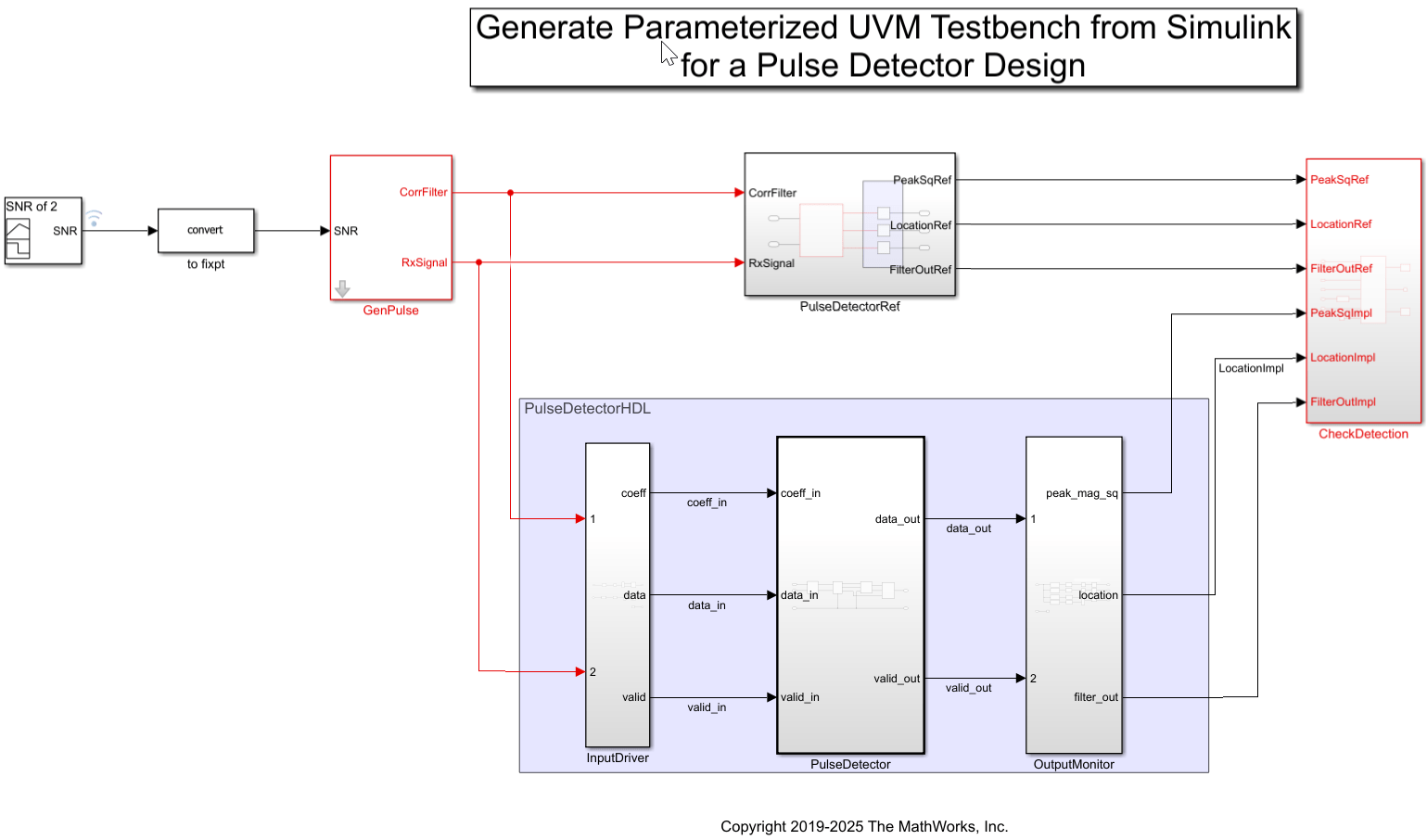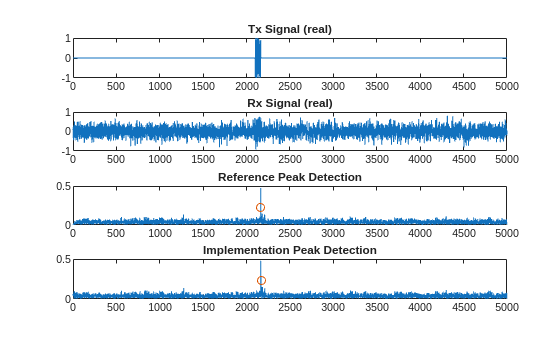# Generate Parameterized UVM Test Bench from Simulink

This example shows how you can develop a design and test bench in Simulink and generate an equivalent simulation for a Universal Verification Methodology (UVM) environment using `uvmbuild`. Related examples show how you can extend this test bench to refine your verification using protocol-specific drivers, constrained random sequences, and parameterized scoreboards.

### Introduction

This example walks you through a top-down design development process of an HDL implementation. In such a workflow, you design a behavioral algorithm in Simulink and test it using surrounding blocks for stimulus generation and results checking. Once the simulation confirms that the design meets its requirements, you deliver any collateral needed to the downstream HDL implementation team. You need to re-verify the HDL implementation meets the requirements as simulated in Simulink as well as any other unique aspects of the design such as protocol interfaces that were not modeled in Simulink.

Ordinarily the hand-off process can be tedious and the source of many errors. The HDL implementation and HDL Design Verification (DV) engineers must:

• Translate written specifications to HDL and testing environments.

• Understand the run-time behavior of the Simulink simulation environment such as how the stimulus is created, processed, and checked.

• Translate the run-time behaviors to SystemVerilog implementations.

• Integrate the stimulus, design, and response checking into a runnable SystemVerilog to confirm that the translated behaviors behave the same as the original Simulink simulation.

• Integrate these main SystemVerilog components into a UVM context in order to allow extending the Simulink testing with DV-authored verification. This extended testing might include randomized testing, SystemVerilog assertions, functional coverage, and code coverage.

Using the HDL Verifier UVM generation capabilities, this hand-off process is automated. The DV engineer gets a verified UVM test environment that matches the testing performed in Simulink and can easily update that environment to meet their downstream verification needs.

### Design and Test in Simulink

Write your algorithm and add a test bench to it. The model consists of typical subsystems for a test bench such as stimulus generation, the design under test (DUT), and response checking.

In this design, the source subsystem creates a random pulse of 64 samples of information embedded at a random location in a 5000 sample frame of noise. It also generates a set of 64 optimal matched filter coefficients for detection of the pulse. The inputs are fed to both the design and the response checker. The response checker verifies that the pulse is detected at the right location in the noisy waveform. Proper operation is confirmed through console output. If the expected power of the detected signal is not within certain limits, an assertion is fired.Simulating the model provides confirmation that in five generated pulses, five are detected. A three paneled figure shows a Tx Signal (the original pulse), an Rx Signal (the pulse embedded in noise), and the filtered output of a reference implementation that shows where a peak is detected. The output signal is delayed by one frame.

```[FrameNum= 0] Peak location=2163.000000, mag-squared=0.280 using global max [FrameNum= 0] Peak detected from impl=2163 error(abs)=0 [FrameNum= 0] Peak mag-squared from impl=0.280, error(abs)=0.000 error(pct)=0.017 [FrameNum= 1] Peak location=2163.000000, mag-squared=0.200 using global max [FrameNum= 1] Peak detected from impl=2163 error(abs)=0 [FrameNum= 1] Peak mag-squared from impl=0.199, error(abs)=0.000 error(pct)=0.190 [FrameNum= 2] Peak location=2163.000000, mag-squared=0.224 using global max [FrameNum= 2] Peak detected from impl=2163 error(abs)=0 [FrameNum= 2] Peak mag-squared from impl=0.223, error(abs)=0.000 error(pct)=0.183 [FrameNum= 3] Peak location=2163.000000, mag-squared=0.200 using global max [FrameNum= 3] Peak detected from impl=2163 error(abs)=0 [FrameNum= 3] Peak mag-squared from impl=0.200, error(abs)=0.000 error(pct)=0.043 [FrameNum= 4] Peak location=2163.000000, mag-squared=0.255 using global max [FrameNum= 4] Peak detected from impl=2163 error(abs)=0 [FrameNum= 4] Peak mag-squared from impl=0.255, error(abs)=0.000 error(pct)=0.031 [FrameNum= 5] Peak location=2163.000000, mag-squared=0.241 using global max [FrameNum= 5] Peak detected from impl=2163 error(abs)=0 [FrameNum= 5] Peak mag-squared from impl=0.241, error(abs)=0.000 error(pct)=0.187 [FrameNum= 6] Peak location=2163.000000, mag-squared=0.241 using global max [FrameNum= 6] Peak detected from impl=2163 error(abs)=0 [FrameNum= 6] Peak mag-squared from impl=0.241, error(abs)=0.000 error(pct)=0.019 [FrameNum= 7] Peak location=2163.000000, mag-squared=0.225 using global max [FrameNum= 7] Peak detected from impl=2163 error(abs)=0 [FrameNum= 7] Peak mag-squared from impl=0.225, error(abs)=0.000 error(pct)=0.032 [FrameNum= 8] Peak location=2163.000000, mag-squared=0.239 using global max [FrameNum= 8] Peak detected from impl=2163 error(abs)=0 [FrameNum= 8] Peak mag-squared from impl=0.239, error(abs)=0.000 error(pct)=0.037 [FrameNum= 9] Peak location=2163.000000, mag-squared=0.225 using global max [FrameNum= 9] Peak detected from impl=2163 error(abs)=0 [FrameNum= 9] Peak mag-squared from impl=0.225, error(abs)=0.000 error(pct)=0.146 [FrameNum= 10] Peak location=2163.000000, mag-squared=0.207 using global max [FrameNum= 10] Peak detected from impl=2163 error(abs)=0 [FrameNum= 10] Peak mag-squared from impl=0.207, error(abs)=0.000 error(pct)=0.134 ```### Generate an Executable UVM Test Bench

Use the `uvmbuild` function to export your design to a UVM environment. The UVM test bench provides structure to the HDL verification process and allows for all of the Simulink test bench components and test cases to be reused by the implementation verification team. Standard component definitions separate the pieces of the environment by their role in the simulation. For this example:

• `PulseDetector` is mapped to the DUT SystemVerilog module

• `GenPulse` subsystem is mapped to the `sequence_item` creation for the Sequencer UVM component

• `CheckDetection` subsystem is mapped to the Scoreboard UVM component.

The generated UVM test bench is shown below:```% Generate a UVM test bench design = 'prm_uvmtb/PulseDetector' sequence = 'prm_uvmtb/GenPulse' scoreboard = 'prm_uvmtb/CheckDetection' uvmbuild(design, sequence, scoreboard) ```

Each of the highlighted pieces of the UVM test bench are implemented by wrapping generated C-code from the Simulink subsystem and calling its entry points using DPI. The following image shows a couple of the function declarations for the PulseDetector subsystem.The SystemVerilog/UVM code determines the timing of the DPI calls. For example, in the PulseDetector SystemVerilog module:

• The "initialize" DPI call is triggered by an "initial" code block.

• The "terminate" DPI call is triggered by a "final" code block.

• The "reset" DPI call is triggered by an active reset signal.

• The "output" and "update" DPI calls are triggered by a rising clock edge where reset is not active and the clock enable is active.### Run the UVM Test Bench

The `uvmbuild` process also generates a script to run a simulation of the UVM test. Scripts are generated for the following simulators:

• Mentor Graphics® Modelsim® and Questa®: run_tb_mq.do

• Synopsys® VCS®: run_tb_vcs.sh

The generated script for ModelSim is shown.Execute the generated script to verify the UVM execution matches the Simulink execution. Because the sequence is parameterized with the SNR input port, its default value will be 0.0 in UVM. In order to properly compare the simulation runs, we need to change its default value to 2.0 (which has a bit value of 0b10_000000), to match Simulink; this can be done via a plusarg which we pass to the script via an environment variable.

```% Clear environment variables that influence the UVM simulation' setenv EXTRA_UVM_SIM_ARGS setenv EXTRA_UVM_COMP_ARGS setenv UVM_TOP_MODULE ```
```% Simulate the UVM test bench using an SNR of 2.0 cd prm_uvmtb_uvmbuild/uvm_testbench/top setenv EXTRA_UVM_SIM_ARGS +SNR_default_inp_val=10000000 ! vsim -do run_tb_mq.do % ModelSim/QuestaSim (gui) ! vsim -c -do run_tb_mq.do % ModelSim/QuestaSim (console) ! ./run_tb_incisive.sh % Incisive (console) ! ./run_tb_xcelium.sh % Xcelium (console) ! ./run_tb_vcs.sh % VCS (console) cd ../../.. ```

The simulation log shows the same diagnostic messages:And the waveform shows the timing of the DUT interface signals. The cursor is placed at a frame boundary and shows the instantaneous update of the matched filter coefficients.##### SupportGet trial now